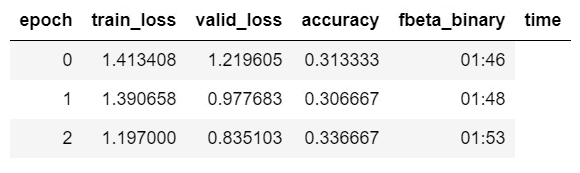# Help using Fbeta_binary

Hello,

I’m trying to print f2 score for a 3 class classification problem. Here’s the Fbeta_binary class (which I found in one of the forum posts). I’ve added this class to metrics.py

``````@dataclass
class Fbeta_binary(Callback):
"Computes the fbeta between preds and targets for single-label classification"
beta2: int = 2
eps: float = 1e-9
clas:int=1

def on_epoch_begin(self, **kwargs):
self.TP = 0
self.total_y_pred = 0
self.total_y_true = 0

def on_batch_end(self, last_output, last_target, **kwargs):
y_pred = last_output.argmax(dim=1)
y_true = last_target.float()
print(last_target)

self.TP += ((y_pred==self.clas) * (y_true==self.clas)).float().sum()
self.total_y_pred += (y_pred==self.clas).float().sum()
self.total_y_true += (y_true==self.clas).float().sum()

def on_epoch_end(self, last_metrics, **kwargs):
beta2=self.beta2**2
prec = self.TP/(self.total_y_pred+self.eps)
rec = self.TP/(self.total_y_true+self.eps)
res = (prec*rec)/(prec*beta2+rec+self.eps)*(1+beta2)
self.metric = res
``````

I call `create_cnn` like this

``````fb0 = Fbeta_binary(beta2=2,clas = 0)
learn = create_cnn(data, models.resnet34,
metrics=[accuracy, fb0])
``````

Alternatively, I’ve also tried the logic mentioned in this notebook - where in I define `Fbeta_binary` class in the notebook and pass it `create_cnn`.

In both cases I don’t see fbeta getting printed in the outputAny idea what might be happening?

Ok, I think i figured it out. Something small and stupid. Had to `return add_metrics(last_metrics, self.metric)`